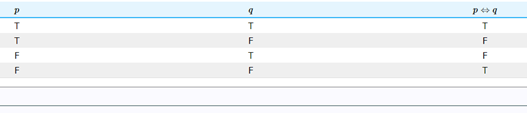# Truth Tables for Biconditionals Worksheets

What Are the Trends of Truth Tables for Biconditionals? In mathematical logic, every statement is either true or false. The logical biconditional statement is also called material biconditional. It logically joins the two statements p and q. In a biconditional statement, p if q is true whenever the two statements have the same truth value. Otherwise, it is false. It is denoted as p ↔ q. In other words, logical statement p ↔ q implies that p and q are logically equivalent. A logic involves the connection of two statements.For better understanding, you can have a look at the truth table above. A logic statement is true if both the statements carry the same meaning. It is true either when bot the statements are true, or both are false. Otherwise, it is false.

• ### Basic Lesson

Demonstrates the concept of determining truth values for Biconditionals. Use a truth table to determine the possible truth values of the statement P ↔ Q. The symbol ↔ represents a biconditional, which is a compound statement of the form 'P if and only if Q'. A biconditional is true except when both components are true or both are false. If they are not the same, it is false.

• ### Intermediate Lesson

Shows students how to determine truth values for Biconditionals. Set up a truth table for the simple statements P and Q.

• ### Independent Practice 1

Contains a mixture of problems using Biconditionals. Students must determine the truth value.

• ### Independent Practice 2

Features truth value questions with assorted concepts. Students concentrate on biconditionals.

• ### Homework Worksheet

Features 6 biconditional problems; students must determine the truth-value.

• ### Skill Quiz

10 truth value questions that include biconditionals. Scoring matrix is provided.

• ### Homework and Quiz Answer Key

Answers for the homework and quiz.